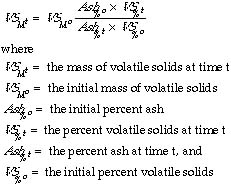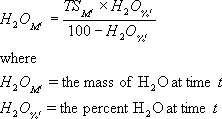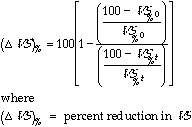## Determining Volatile Solids and Moisture Losses

Tom Richard

In large and even medium sized composting systems it can be difficult to directly measure the mass of substrate once the composting process has started, so it is difficult to determine the mass that has been degraded to CO2 and H2O. For a completely mixed reactor, the ash and volatile solids percents of a sample and the original mass data can be used to calculate the volatile solids mass remaining using the following equation:Note that for any sample, ash and volatile solids are related by:

VS%= 100-Ash%

The reduction in volatile solids concentration is gradual and often small, so replicated samples should be ashed to insure a statistically representative result.

You can combine this result with moisture data to determine the moisture loss from the compost. First, calculate the VS loss, and then subtract this DVS from the total dry solids (TS) in the system to get the TS mass at time t (note that the ash fraction of TS is conserved, so the loss of TS is equal to the loss of VS):At any point in time, the mass of water remaining in the compost can be calculated from:In addition to the mass changes in volatile solids or water, one can also calculate the percent reductions in VS or H2O using the following formulas:andAcknowledgement:

Many thanks to Mr. Vladimir Knezevic for correcting an error in the formula for the percent reduction in the mass of water. The corrected formula was posted on this page November 17, 2000.Cornell Composting Science & Engineering Composting in Schools Resources

Cornell Waste Management Institute © 1996
Cornell University
Ithaca, NY 14853
607-255-1187
cwmi@cornell.edu